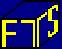Case Objective

To understand the time value of money; to understand the cash flows from coupon and zero coupon bonds; to apply the discounting formula to value such bonds.

Key Concepts

Time value of money; discounting; determining bond prices given interest rates.

Case description

The first bond is a coupon bond with a coupon interest rate equal to 20% per annum, the second is a zero-coupon bond.  Both bonds have a face value equal to 100.  The cash flow from each bond at the end of each period is shown in the table below.

The interest rate is fixed at 25% per annum.  If you have any cash left at the end of a period, you will receive interest on the cash at 25% at year end.  You are also allowed borrow cash (at 25%). That is, the borrowing and lending rate of interest is the same in B01.

Interest is earned if your end of period cash balance, before any coupon payments are received or paid, is positive as follows:

Interest earned = End of period cash (before coupon payments) * 0.25.

If you have borrowed cash so that your end of period cash balance is negative (before coupon payments are received or paid) then the above interest calculation is subtracted from your cash balance.

You can buy and sell any bond and short sell any bond.  That is, unlike real assets, short selling means that you can sell bonds that you do not currently own!  This is possible in a financial market because you are legally obligated to cover your short position by paying any coupon payments or face amount at the appropriate time.  For example, if you hold a short position in the zero coupon bond at the end of period 3 then the face amount of 100 times the number of bonds you are short, is automatically deducted from your closing cash balance (after interest is calculated).  That is, you will earn interest at the money market rate of 25% on funds generated from your short selling activities first before you have to cover any cash payments required from your short position.  The same applies to the coupon bond except that given it has a three period life you will need to cover the coupon payments at the end of each period and both the face amount and coupon payment at the end of period 3 if you had a short position in each trading period.

Summary:  The sequence of adjustments to end of period cash balance is:

First, interest earned or owed is computed

Second, coupon payments and/or face amount payments are added to (or subtracted) from your end of period cash balance net of interest payments if you are long (short) a bond.

All bond prices in this case are determined by the traders in the market.  That is, all trades will take place at bids and asks that either you or another trader in the market submits during a trading period.

Case Data

The cash flows for the two bonds are shown below.

 Payout at end of Period 1 Payout at end of Period 2 Payout at end of Period 3 Cp Bond 20 20 120 Zero Cp 0 0 100